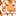# New to Qlik Sense

If you’re new to Qlik Sense, start with this Discussion Board and get up-to-speed quickly.

cancel
Showing results for
Did you mean:Creator

## Check data for info

Hi,

I have data from a hospital. My data have a row per stay at a department in the hospital with the name of the department and an ID for the hospital-stay. The same patient can have stays in multiple departments during the same hospital-stay.

I need to do a check of data to get information from all the patients different stays  at departments during their hospital-stay.

I want to know if patients only have a stay at only one specific department. Or if the patients only have  stays at two specific departments. And finally if the patients have stays at two specific departments and at least one stay in 3. department.

I have tried to illustrate it  below. The red text is the info that I am searching for. I would like to find a solution for use in load script.

Anybody that can help?

 Id Department Wanted info: 1 A Only A 2 A Only A and B 2 B Only A and B 3 A A, B and other department 3 B A, B and other department 3 C A, B and other department 3 D A, B and other department 4 E Other
Labels (1)
• ### Script

1 Solution

Accepted SolutionsSpecialist

Hi, if you not have dozens of departments here you can try something like this maybe:

main:
[Id, Department
1,A,
2,A,
2,B,
3,A,
3,B,
3,C,
3,D,
4,E ];

temp:
count(if(Department='A',1)) as A,
count(if(Department='B',1)) as B,
count(if(Department='C',1)) as C,
count(if(Department='D',1)) as D,
count(if(Department='E',1)) as E
Resident main
group by Id;

left join (main)
Id,
if( A>0 and B=0 and C=0 and D=0 and E=0, 'Only A',
if( A=0 and B>0 and C=0 and D=0 and E=0, 'Only B',
if( A>0 and B>0 and C=0 and D=0 and E=0, 'Only A and B',
if( A>0 and B>0 and (C>0 or D>0 or E>0), 'A, B and other',
if( A=0 and B=0 and (C>0 or D>0 or E>0), 'Other', 'CHECK'))))) as wanted_info
resident temp;

drop table temp;

3 RepliesCreator
Author

Anybody that can help here ?

I have tried to use the p and e function in set analysis. But I cant get that to work. And I prefer a solution in script editor.Specialist

Hi, if you not have dozens of departments here you can try something like this maybe:

main:
[Id, Department
1,A,
2,A,
2,B,
3,A,
3,B,
3,C,
3,D,
4,E ];

temp:
count(if(Department='A',1)) as A,
count(if(Department='B',1)) as B,
count(if(Department='C',1)) as C,
count(if(Department='D',1)) as D,
count(if(Department='E',1)) as E
Resident main
group by Id;

left join (main)
Id,
if( A>0 and B=0 and C=0 and D=0 and E=0, 'Only A',
if( A=0 and B>0 and C=0 and D=0 and E=0, 'Only B',
if( A>0 and B>0 and C=0 and D=0 and E=0, 'Only A and B',
if( A>0 and B>0 and (C>0 or D>0 or E>0), 'A, B and other',
if( A=0 and B=0 and (C>0 or D>0 or E>0), 'Other', 'CHECK'))))) as wanted_info
resident temp;

drop table temp;Creator
Author

Hi @justISO

It works perfect,

thank you 😊Tags
Community Browser